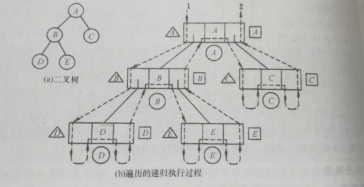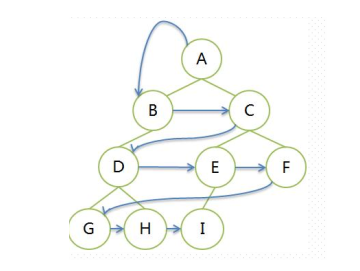# 二叉树的遍历

## 递归遍历

【注】“序”指的是遍历过程中根节点何时被访问。

1.先序遍历

（1）访问根结点；
（2）先序遍历左子树；
（3）先序遍历右子树；

``````void PreOrder(BiTree T){
if(T != NULL){
visit(T);				//访问根节点
PreOrder(T->lchild);	//递归遍历左子树
PreOrder(T->rchild);	//递归遍历右子树
}
}
``````

1.中序遍历

（1） 中序遍历左子树；
（2）访问根结点；
（3） 中序遍历右子树；

``````void InOrder(BiTree T){
if(T != NULL){
InOrder(T->lchild);		//递归遍历左子树
visit(T);				//访问根节点
InOrder(T->rchild);		//递归遍历右子树
}
}
``````

1.后序遍历

（1）后序遍历左子树；
（2）后序遍历右子树；
（3）访问根结点；

``````void PostOrder(BiTree T){
if(T != NULL){
PostOrder(T->lchild);	//递归遍历左子树
PostOrder(T->rchild);	//递归遍历右子树
visit(T);		//访问根节点
}
}
``````## 非递归遍历

1.先序遍历

``````void PreOrderTraverse(BinTree b)
{
InitStack(S);						//初始化创建栈
BinTree p=b;						//p为工作指针
while(p||!isEmpty(s))
{
while(p)						//到最左下的孩子
{
printf(" %c ",p->date);		//先序先遍历结点
Push(S,p);					//入栈
p=p->lchild;
}
if(!isEmpty(s))				//在栈不为空的情况下，左孩子为空，弹出该结点，遍历右孩子
{
p=Pop(s);
p=p->rchild;
}
}
}
``````

``````void InOrderTraverse(BinTree b)
{
InitStack(S);				//初始化创建栈
BinTree p=b;				//p为工作指针
while(p||!isEmpty(s))
{
while(p)
{
Push(S,p);			//中序现将结点进栈保存
p=p->lchild;
}						//遍历到左下角尽头再出栈访问
if(!isEmpty(s))			//在栈不为空的情况下，左孩子为空，弹出该结点，遍历右孩子
{
p=Pop(s);
printf(" %c ",p->data);
p=p->rchild;		//遍历右孩子
}
}
}
``````

``````void PostOrderTraverse(BinTree b)
{
InitStack(S);						//初始化创建栈
BinTree p=b, r=NULL;				//p为工作指针,辅助指针r
while(p||!isEmpty(s))
{
if(p)							//从根节点到最左下角的左子树都入栈
{
Push(S,p);					//中序现将结点进栈保存
p=p->lchild;
}
else
{
GetTop(S,p);					//取栈顶，注意！不是出栈！
if(p->rchild&&p->rchild!=r)		//右子树还没有访问并且右子树不空，第一次栈顶
{
p=p->rchild;				//进入右子树
}
else							//右子树已经访问或为空，接下来出栈访问结点，第二次栈顶
{
p=Pop(s);
printf(" %c ",p->data);
r=p;						//指向访问过的右子树结点
p=NULL;						//使p为空继续访问栈顶
}
}
}
}
``````

## 层序遍历``````void LevelOrder(BiTree T){
InitQueue(Q);				//初始化辅助队列
BiTree p;
EnQueue(Q,T);				//将根节点入队;
while(!IsEmpty(Q)){			//队列不为空则循环
DeQueue(Q,p)				//对头结点出队
visit(p);					//访问出队结点
if(p->lchild != NULL)
EnQueue(Q,p->lchild);	//左孩子不空，左子树根节点入队
if(p->rchild != NULL)
EnQueue(Q,p->rchild);	//右孩子不空，右子树根节点入队
}
}
``````